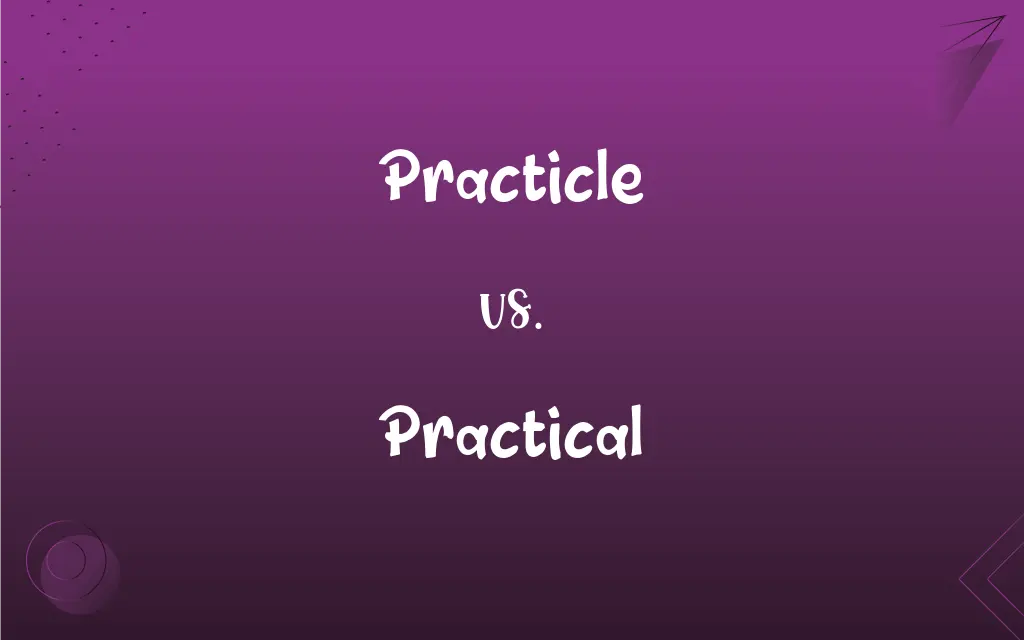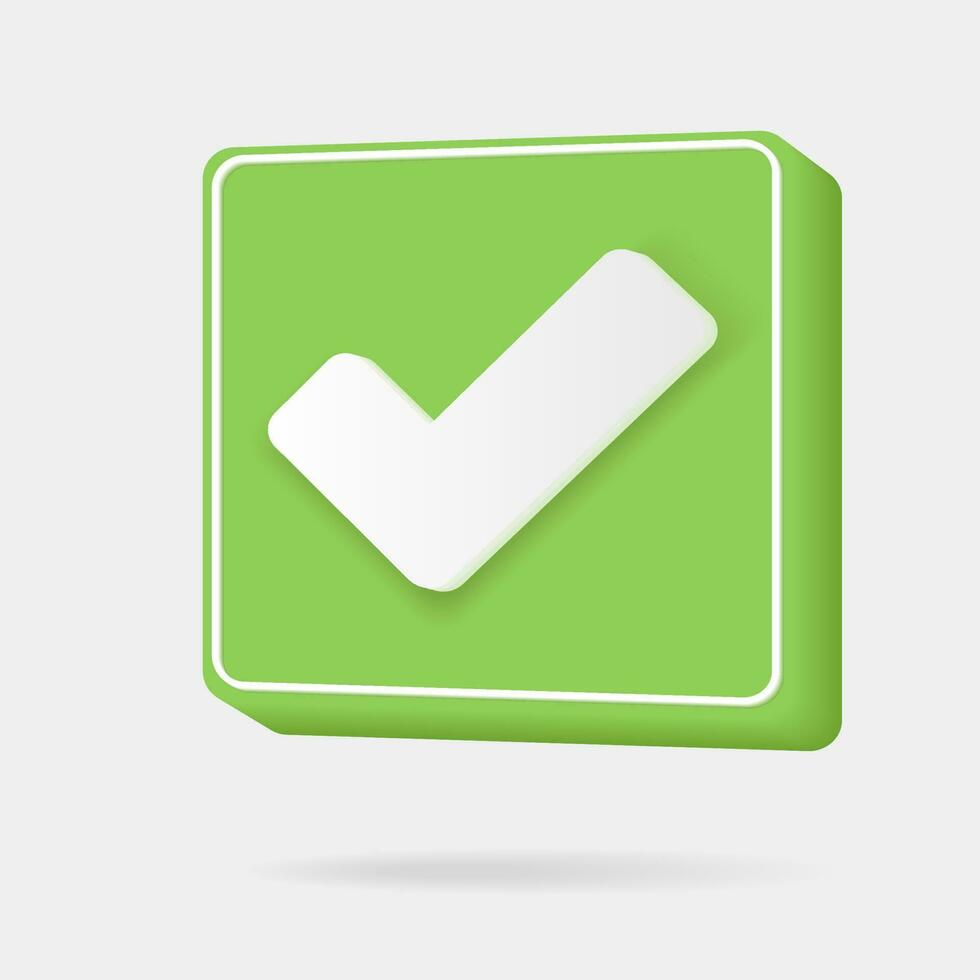# Practicle vs. Practical: What's the Difference?Edited by Huma Saeed || By Sawaira Riaz || Updated on October 22, 2023
"Practicle" is incorrect, while "Practical" is the correct spelling, referring to something effective, applicable, or pragmatic in actual situations.## Which is correct: Practicle or Practical

How to spell Practical?### Practicle is Incorrect## Key Differences

Remember "practical" like "practical joke," both correctly spelled and commonly used together.
"Practical" ends in "-cal" like other adjectives, including "logical" and "theoretical."
Think of "practical" as relating to practice, and practice makes perfect, so no “e” in “practical.”
Recall "practical" contains "act," as it's about taking action, not "ice" which is static and cold.
Associate "practical" with "practicum," both pertaining to hands-on experience and sharing the same root.

## Practicle and Practical Definitions

#### Practicle

Practicle is an incorrect spelling of Practical.

#### Practical

"Practical" involves considering the realities and facts for decision-making.

#### Practical

"Practical" can describe skills or knowledge acquired by hands-on experience.
Carpentry is a practical skill.

#### Practical

"Practical" refers to an approach that is pragmatic or realistic.
A practical solution to hunger is food distribution.

#### Practical

"Practical" means designed for implementation; concerned with the practical aspect.
The invention had many practical applications.

#### Practical

"Practical" means being useful or suited for actual use or action rather than theory.
Practical knowledge is gained through experience.

#### Practical

Of, relating to, governed by, or acquired through practice or action, rather than theory or speculation
Gained practical experience of sailing as a deck hand.

#### Practical

Manifested in or involving practice
Practical applications of calculus.

#### Practical

Capable of or suitable to being used or put into effect; useful
Practical knowledge of Japanese. See Usage Note at practicable.

#### Practical

Concerned with the production or operation of something useful
Woodworking is a practical art.

#### Practical

Having or showing good judgment; sensible
If we're practical, we can do the job quickly.

#### Practical

Being actually so in almost every respect; virtual
The snowstorm was a practical disaster.

#### Practical

Relating to, or based on, practice or action rather than theory or hypothesis.
Jack didn't get an engineering degree, but has practical knowledge of metalworking.
Modern engineering applies science to practical problems.

#### Practical

Being likely to be effective and applicable to a real situation; able to be put to use
Jack's knowledge has the practical benefit of giving us useful prototype parts.

#### Practical

Of a person, having skills or knowledge that are practical
All in all, Jack's a very practical chap.

#### Practical

Of a prop: having some degree of functionality, rather than being a mere imitation.

#### Practical

(film) Light fixtures used for set lighting and seen in the frame of a shot as part of the scenery.
Practical lighting can help sell the illusion that the film is a real situation.

#### Practical

(British) A part of an exam or series of exams in which the candidate has to demonstrate their practical ability

#### Practical

(theater) A prop that has some degree of functionality, rather than being a mere imitation.

#### Practical

(film) A light fixture used for set lighting and seen in the frame of a shot as part of the scenery.

#### Practical

Laboratory experiment, test or investigation

#### Practical

Of or pertaining to practice or action.

#### Practical

Capable of being turned to use or account; useful, in distinction from ideal or theoretical; as, practical chemistry.

#### Practical

Evincing practice or skill; capable of applying knowledge to some useful end; as, a practical man; a practical mind.

#### Practical

Derived from practice; as, practical skill.

#### Practical

Concerned with actual use or practice;
He is a very practical person
The idea had no practical application
A practical knowledge of Japanese
Woodworking is a practical art

#### Practical

Guided by practical experience and observation rather than theory;
A hardheaded appraisal of our position
Completely practical in his approach to business
Not ideology but pragmatic politics

#### Practical

Being actually such in almost every respect;
A practical failure
The once elegant temple lay in virtual ruin

#### Practical

Having or put to a practical purpose or use;
Practical mathematics
Practical applications of calculus

## FAQs

#### What is the root word of Practical?

The root word is the Greek "praktikos," meaning "fit for action."

#### Which preposition is used with Practical?

Prepositions like "for," "in," and "with" can be used with "practical."

#### What is the singular form of Practical?

"Practical" is an adjective and doesn't have a singular or plural form.

#### What is the verb form of Practical?

"Practical" is an adjective; its related verb can be "practice" or "practise" (British English).

#### What is the pronunciation of Practical?

Practical is pronounced as /ˈpræktɪkəl/.

#### What is the plural form of Practical?

"Practical" is an adjective and doesn't have a singular or plural form.

#### Which conjunction is used with Practical?

Standard conjunctions like "and" or "but" can be used with "practical."

No, "practical" is not an adverb, but there is an adverbial form, "practically."

#### Which vowel is used before Practical?

The vowel "a" is used, as in "a practical."

#### Why is it called Practical?

It's called "practical" because it originates from the late Latin word "practicalis," meaning "relating to practice," and it denotes applicability to real-life situations.

#### How many syllables are in Practical?

There are three syllables in "practical."

#### What is the opposite of Practical?

The opposite could be "impractical" or "theoretical."

#### Is Practical a vowel or consonant?

"Practical" is a word, consisting of both vowels and consonants.

#### Is the word Practical imperative?

No, "practical" is not imperative; it's an adjective.

#### What is a stressed syllable in Practical?

The stress is on the first syllable: PRAC-ti-cal.

#### What is another term for Practical?

Another term could be "pragmatic" or "functional."

#### What is the third form of Practical?

N/A - "Practical" is an adjective and doesn’t have different forms like verbs.

#### Is Practical an abstract noun?

No, "practical" is an adjective, not a noun.

#### How is Practical used in a sentence?

"He has a practical approach to solving problems, focusing on realistic solutions."

#### Which article is used with Practical?

Both "a" and "the" can be used with "practical," depending on context.

#### Is Practical a negative or positive word?

It is generally considered positive, emphasizing usefulness and pragmatism.

#### Is Practical a countable noun?

"Practical" is an adjective and does not have a countable form.

#### Is Practical a collective noun?

No, "practical" is not a collective noun.

#### Is the Practical term a metaphor?

No, "practical" is not typically used as a metaphor. It is a descriptive term.

#### How do we divide Practical into syllables?

It's divided as prac-ti-cal.

#### Which determiner is used with Practical?

Determiners like "the," "this," "my," etc., can be used with "practical."

#### What is the first form of Practical?

"Practical" is an adjective and doesn't have forms like verbs do. Its basic form is "practical."

#### What is the second form of Practical?

N/A - "Practical" is an adjective and doesn’t have different forms like verbs.